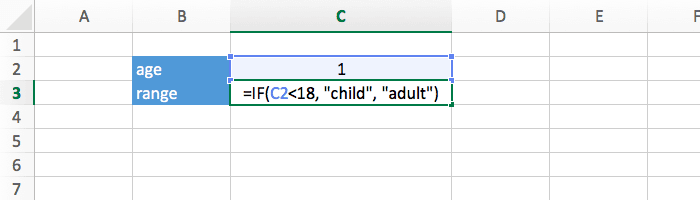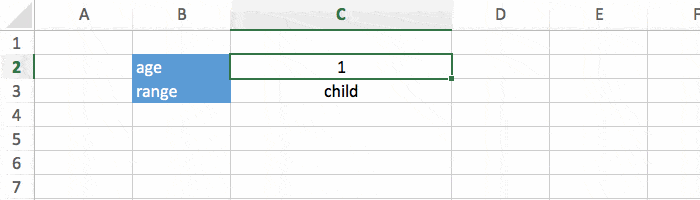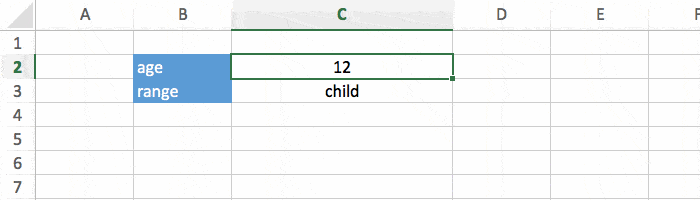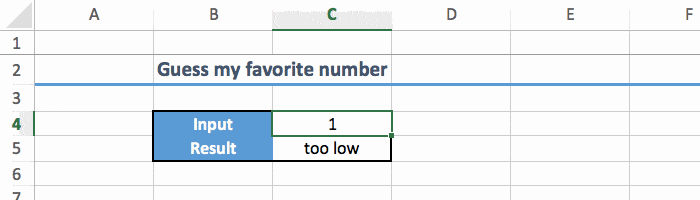# Excel: Understand IF, Nested IF, and IFS Functions

In Microsoft Excel, the IF function is simple yet incredibly powerful. In this short tutorial we will see how to use IF, nested IF, and the new IFS functions.

## IF Function

The IF function tests a condition, and then reacts differently depending on whether the test was true or false. The function itself looks like this:

`=IF(test, value-if-true, value-if-false)`

Let's try to use it in an example. We have the age of someone, and we want to display "child" if he is less than 18 years old, or "adult" if he is older. This can be done with an IF, like this:

• test: AGE<18.
• value-if-true: "child".
• Formula: `=IF(AGE<18, "child", "adult")`.

Just replace AGE by a cell that contains the actual age. In the spreadsheet below it's C2.And when we edit the age, the text is updated properly.That's nice, but we can do even more powerful stuff with nested IF.

## Nested IF Functions

Let's continue our previous example, but this time we want to have 3 age groups: "child" when less than 18, "adult" when less than 80, and "old" otherwise. You cannot do that with a single IF, 2 are needed:

`=IF(AGE<18, "child", IF(AGE<80, "adult", "old"))`

The first IF tests whether or not the person is a child, and if not, the second IF will check if he is an adult or an old person.This works fine, but if you start nesting more than 2 IF, thing will start to get hard to read. That's why you might be interested in the IFS function.

## IFS Function

IFS is a brand new function that does the same thing as nested IF, but in a clearer way. It looks like this:

`=IFS(test1, value-if-true, test2, value-if-true)`

And you can put as many tests as you'd like. Note that this function only works in Excel 2016 and above. If you try to use IFS in an older version, you'll see the error #NAME?.

With IFS, our previous example can be re-written like this:

• Before: `=IF(AGE<18, "child", IF(AGE<80, "adult", "old"))`
• After: `=IFS(AGE<18, "child", AGE<80, "adult", AGE>=80, "old")`

Both lines do the exact same thing, but the second version is a little easier to read. That's why I recommend using IFS instead of nested IF.

## Logical Tests

Here's the list of all the logical tests you can do in an IF with examples:

• Equal to: A1=A2.
• Higher than: A1>A2.
• Higher or equal than: A1>=A2.
• Less than: A1<A2.
• Less or equal than: A1<=A2.
• Not equal to: A1<>A2.

This is pretty straightforward, except the "not equal to" that combines "higher than" and "less than" at the same time.

## Bonus

Here's a little game that I made, where players have to guess my favorite number. How would you create that?Here's the answer below. As you can see, you can make a simple game in excel in just a line of code :-)

`=IFS(C4<42, "too low", C4>42, "too high", C4=42, "you win!")`

## Conclusion

By now you should have a good understanding of the differences between all the IF functions and how to use them.

FYI, here's a quick summary of what we covered:

• `=IF(test, true, false)`
• `=IF(test1, true, IF(test2, true, false))`
• `=IFS(test1, true, test2, true, test3, true)`